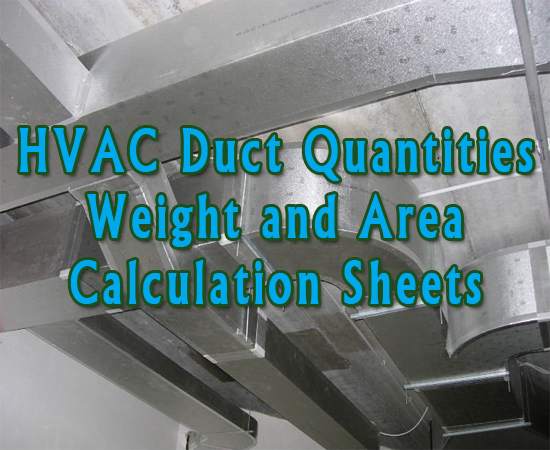HVAC Duct Quantity, Weight and Area Calculation Sheets

# HVAC Duct Quantity, Weight and Area Calculation Sheets

Air Duct quantity can be estimated in sq. meters or kilograms. These excel sheets will enable you to calculate or estimate the duct weight or the duct surface area.

Before you get in to the estimation you have to consider two things in the drawing sheet

1) units of height and width of a duct. Mostly it would be in millimeters.
2) scale of the drawing sheet.

To calculate the rectangular duct area, we need height, width and length.

when the quantity take off is completed, take 10% extra of duct this will resolve the quantity assumption .HVAC Duct Quantity, Weight and Area Calculator

### The Excel sheet below calculate the HVAC duct quantity:

• Duct Weight
• Duct Surface Area
• Contains all ASHRAE and Carrier tables

1.1.2.